# Short Tutorial on PSpice

Spice is a program developed by the EE Department at the University of California at Berkeley for computer simulation of analog circuits.  In its original form you tell Spice what elements are in the circuit (resistors, capacitors, etc.), and then enter the circuit diagram as an ASCII file showing what nodes each element is connected to.  Every node is assigned a number, and there is always a ground node, which is Number 0.  You then tell Spice what information you want -- bias conditions, frequency response, and/or transient response.  Spice does the circuit analysis and puts out an ASCII file with the information.

Using Spice is not very intuitive to use because the input is an ASCII file rather than a circuit diagram, and the output is another ASCII file rather than a graph.  Several companies have developed graphical user interfaces for Spice, which make it much easier to use.  One of the most popular is PSpice.  PSpice provides a free student version of its program which can be downloaded from www.pspice.com.

To use PSpice, start with the PSpice Schematics program.  When you start up you will get a screen which looks like this: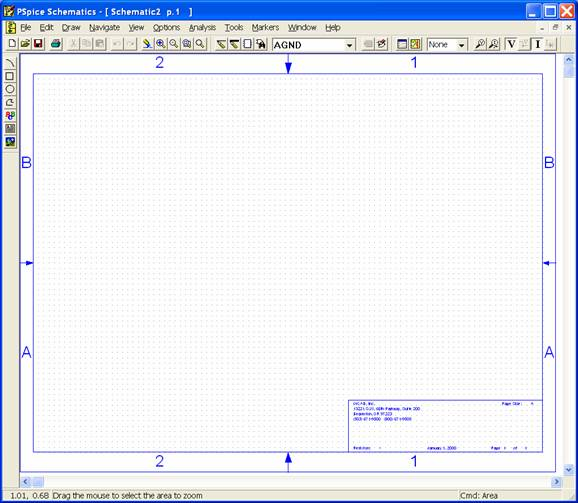To put in a component, use the Draw drop-down menu, and select Get new part (or use the shortcut Ctrl-G).  This will bring up a dialog box which will allow you to select pats from libraries.  If the part you want is not on the list, try another library – parts such as transistors will probably be in eval.slb, while things such as voltage sources will be in analog.slb.  Select the part you want and place it on the schematic: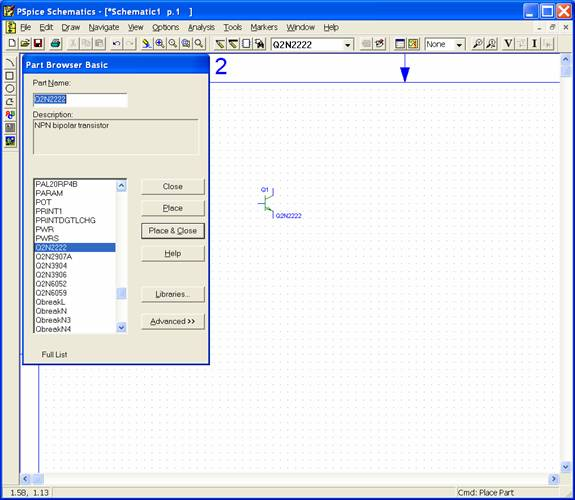Continue placing the components you need.  (If need a component of the same type as one you have already placed, you can use the Draw – Place Part (Ctrl-P) shortcut.)  You can rotate an object by clicking on it to highlight it, then use EditRotate (or Ctrl-R).  You can change the value of a component by double-clicking on the component value, and entering a new value.  You can connect components together by placing wires – Draw – Wire (or Ctrl-W).  Be sure to place an analog ground (AGND).  Use the component VDC for DC power supplies, and VAC for signal sources.  When done you will have something which looks like this: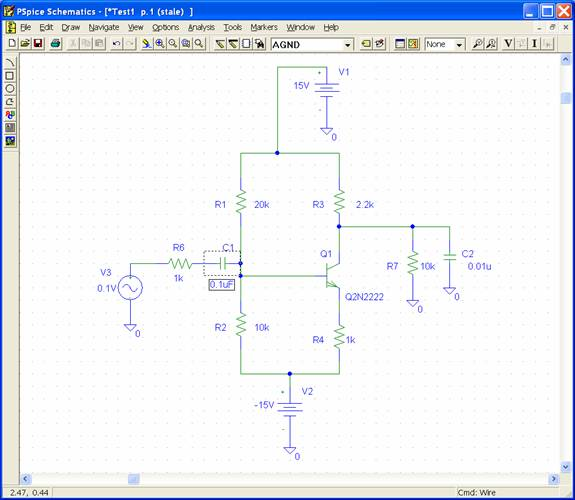Be sure to save the file, then go to Analysis – Setup.   Here you will tell PSpice what you want it to do.  Always select Bias Point Detail.  In this case we will also select AC Sweep which will give the frequency response of the circuit.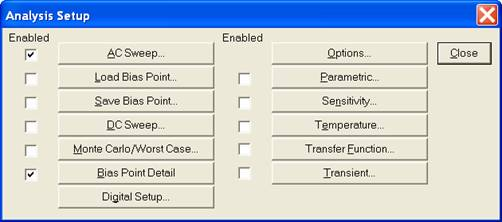Click on AC Sweep to tell what frequency range you want to use: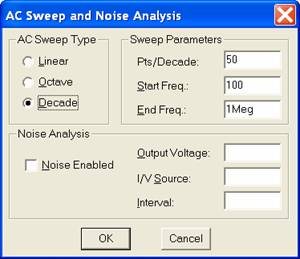Here we will cover the frequencies from 100 Hz to 1 MHz.  (Note:  Use Meg for 106.  If you use M, PSpice will interpret this as milli (10-3).)  Now choose Analysis – Simulate and PSpice will run, and pop up an analysis window: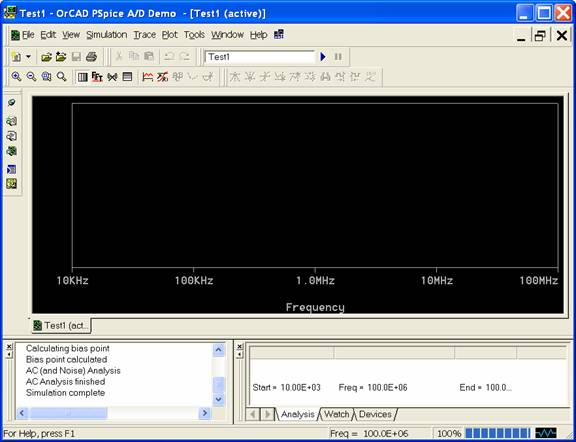In this window choose Trace – Add Trace.  Since we’re interested in the gain of the circuit, we want to plot the output voltage divided by the input voltage.  The output voltage is the voltage at the collector of Q1, and the input voltage is the voltage at the + terminal of V3, so we plot VC(Q1)/V(V3+).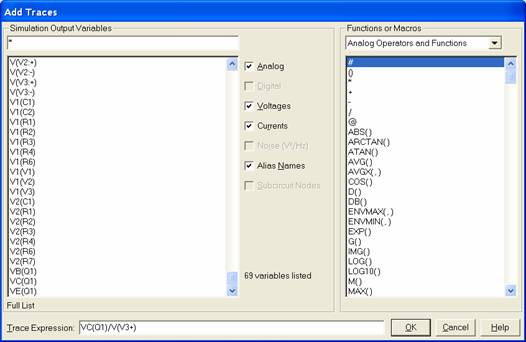We now see the frequency response plot: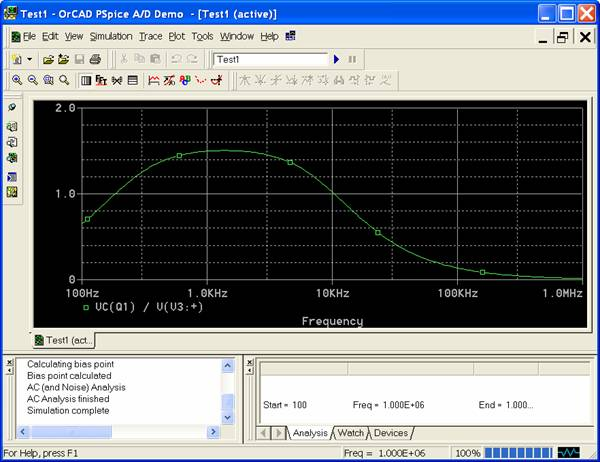We see the circuit has a gain of about 1.5 at a frequency of 1 kHz.  The theoretical value is about (RL || RC)/RE, or 1.8.

To see the bias voltages and currents, you can look at the ASCII output file.  However, it is easier to go back to the Schematic program and select Analysis – Display Results on Schematic and then Enable Voltage Display and/or Enable Current Display.  Here is the schematic with the bias voltages displayed: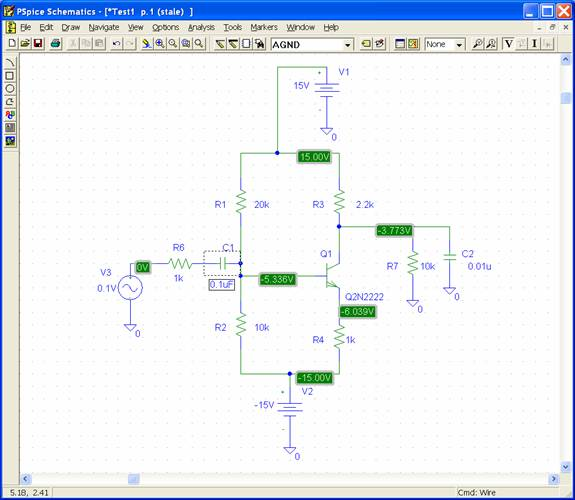It is instructive to look at the ASCII output file.  Here is part of it:

Q_Q1         \$N_0002 \$N_0001 \$N_0003 Q2N2222

R_R4         \$N_0004 \$N_0003  1k

R_R2         \$N_0004 \$N_0001  10k

C_C2         \$N_0002 0  0.01u

V_V2         \$N_0004 0 -15V

R_R6         \$N_0006 \$N_0005  1k

V_V3         \$N_0006 0 DC 0V AC 0.1V

V_V1         \$N_0007 0 15V

R_R1         \$N_0001 \$N_0007  20k

R_R3         \$N_0002 \$N_0007  2.2k

R_R7         0 \$N_0002  10k

C_C1         \$N_0005 \$N_0001  0.1uF

This is the type of file Spice needs – it shows that Q1 is a 2N2222 transistor, and its collector is connected to Node 2, its base to Node 1, and its emitter to Node 3.

Later on in the output file we find the specifications for the 2N2222 transistor:

Q2N2222

NPN

IS   14.340000E-15

BF  255.9

NF    1

VAF   74.03

IKF     .2847

ISE   14.340000E-15

NE    1.307

BR    6.092

NR    1

RB   10

RC    1

CJE   22.010000E-12

MJE     .377

CJC    7.306000E-12

MJC     .3416

TF  411.100000E-12

XTF    3

VTF    1.7

ITF     .6

TR   46.910000E-09

XTB    1.5

CN    2.42

D     .87

The standard Spice model assumes the 2N2222 has a b of 255.9.  You can edit the transistor model if you want to use a different value of b.

We can also see the bias voltages at the nodes:

NODE   VOLTAGE                   NODE   VOLTAGE

(\$N_0001)   -5.3359              (\$N_0002)   -3.7732

(\$N_0003)   -6.0390              (\$N_0004)  -15.0000

(\$N_0005)    0.0000              (\$N_0006)    0.0000

(\$N_0007)   15.0000

Here is the schematic for the RF amplifier circuit of Lab 12: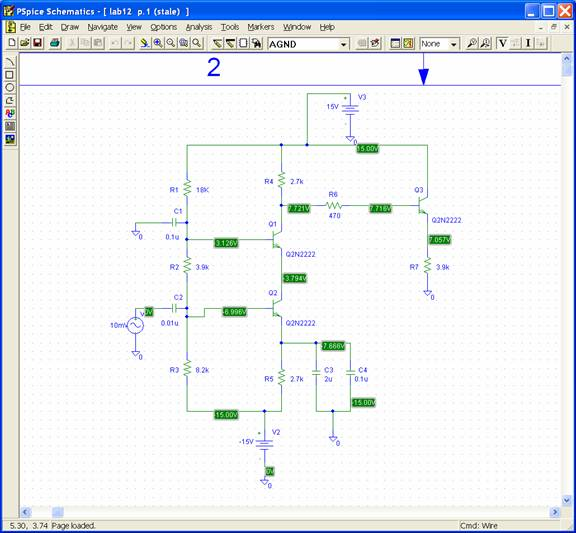and the frequency response shows the passband gain is about 280: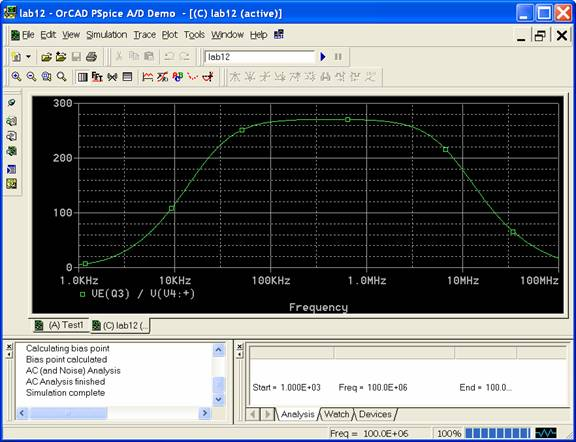For some circuits the transient response is more important.  For example, consider the CMOS inverter: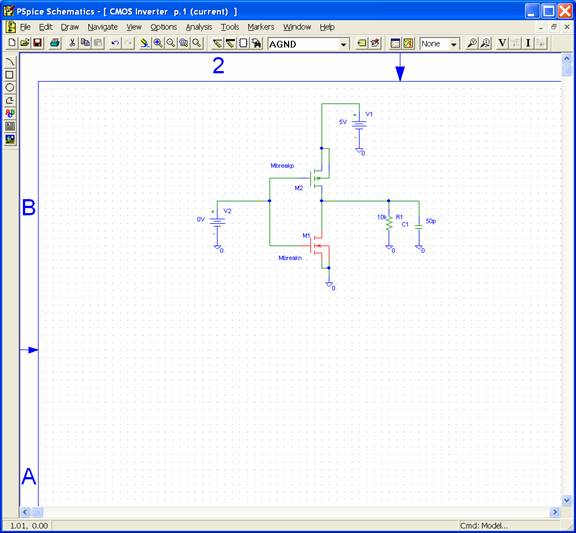The MOSFET models are located in the breakout.slb library, under the names Mbreakn (NMOS) and Mbreakp (PMOS).  We now need to define the parameters of the MOSFETS:  highlight the NMOS transistor and select Edit – Model:Select Edit Instance Model (Text):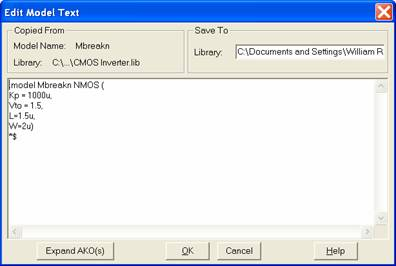and enter appropriate values for  the parameters.  Here we have

kn’ = 100 mA/V2, Vt = 1.5 V, L = 1.5 mm, and W = 2 mm.

Do the same for the PMOS transistor.

Now we will do a DC sweep rather than an AC sweep.  Choose Analysis – Setup, then select DC Sweep.  Sweep source V2 from 0V to 5V at 0.01V increments: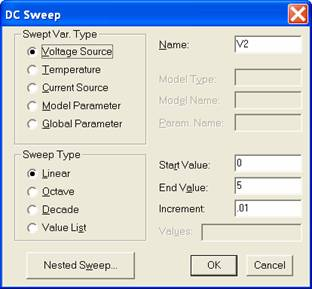In the Graph window, choose Trace – Add Trace, and add V(R1:1), the voltage at the load resistor: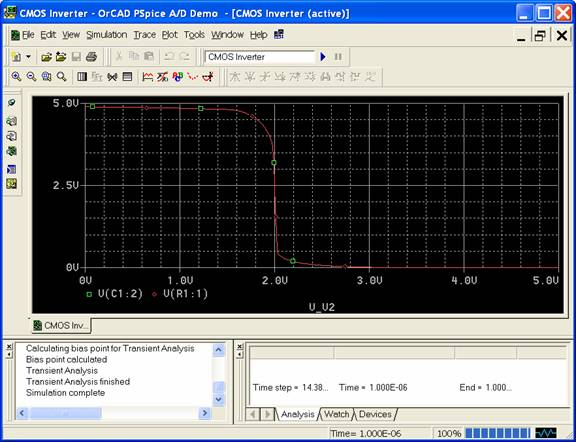and we have the voltage transfer function for the inverter.

Let’s look at the rise and fall times of the circuit.  Change the input voltage source to a VPWL (piece-wise linear source).  Double-click on the source, and enter the shape of the source voltage: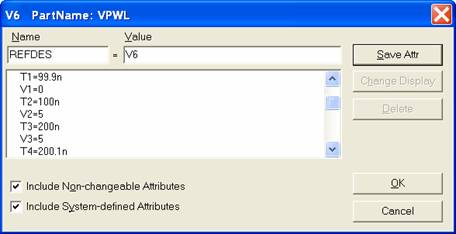Have the source change from 0 V at 99.9 ns to 5 V at 100 ns, then from 5 V at 200 ns to 0 V at 200.1 ns.  This will give a pulse input with a 0.1 ns rise time.  Under Analysis – Setup, choose Transient, and simulate the circuit.  In the graph window plot V(R1:1):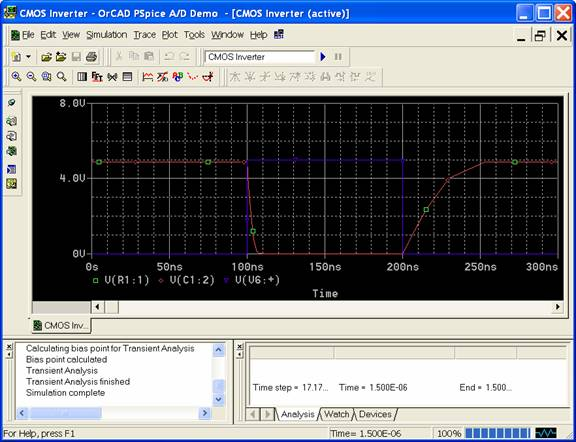and we can see the rise and fall times of the CMOS inverter.